Download Presentation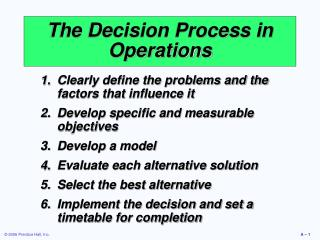The Decision Process in Operations

# The Decision Process in Operations - PowerPoint PPT Presentation

The Decision Process in Operations. Clearly define the problems and the factors that influence it Develop specific and measurable objectives Develop a model Evaluate each alternative solution Select the best alternative Implement the decision and set a timetable for completion.I am the owner, or an agent authorized to act on behalf of the owner, of the copyrighted work described.
Download Presentation## The Decision Process in Operations

An Image/Link below is provided (as is) to download presentation

Download Policy: Content on the Website is provided to you AS IS for your information and personal use and may not be sold / licensed / shared on other websites without getting consent from its author.While downloading, if for some reason you are not able to download a presentation, the publisher may have deleted the file from their server.

- - - - - - - - - - - - - - - - - - - - - - - - - - E N D - - - - - - - - - - - - - - - - - - - - - - - - - -
Presentation Transcript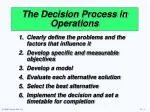The Decision Process in Operations

Clearly define the problems and the factors that influence it

Develop specific and measurable objectives

Develop a model

Evaluate each alternative solution

Select the best alternative

Implement the decision and set a timetable for completion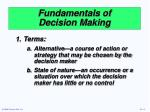Fundamentals of Decision Making
• Terms:
• Alternative—a course of action or strategy that may be chosen by the decision maker
• State of nature—an occurrence or a situation over which the decision maker has little or no control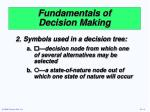Fundamentals of Decision Making
• Symbols used in a decision tree:
• —decision node from which one of several alternatives may be selected
• —a state-of-nature node out of which one state of nature will occur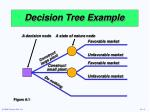A decision node

A state of nature node

Favorable market

Unfavorable market

Construct large plant

Favorable market

Construct small plant

Unfavorable market

Do nothing

Decision Tree Example

Figure A.1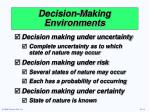Decision-Making Environments
• Decision making under uncertainty
• Complete uncertainty as to which state of nature may occur
• Decision making under risk
• Several states of nature may occur
• Each has a probability of occurring
• Decision making under certainty
• State of nature is known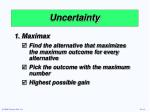Uncertainty
• Maximax
• Find the alternative that maximizes the maximum outcome for every alternative
• Pick the outcome with the maximum number
• Highest possible gain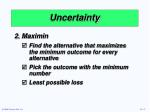Uncertainty
• Maximin
• Find the alternative that maximizes the minimum outcome for every alternative
• Pick the outcome with the minimum number
• Least possible loss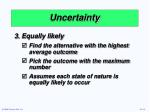Uncertainty
• Equally likely
• Find the alternative with the highest average outcome
• Pick the outcome with the maximum number
• Assumes each state of nature is equally likely to occur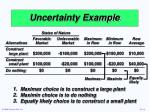States of Nature

Favorable Unfavorable Maximum Minimum Row

Alternatives Market Market in Row in Row Average

Constructlarge plant\$200,000 -\$180,000 \$200,000 -\$180,000 \$10,000

Constructsmall plant\$100,000 -\$20,000 \$100,000 -\$20,000 \$40,000

Do nothing\$0 \$0 \$0 \$0 \$0

Maximax

Maximin

Equally likely

Uncertainty Example

Maximax choice is to construct a large plant

Maximin choice is to do nothing

Equally likely choice is to construct a small plant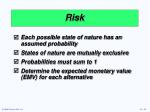Risk
• Each possible state of nature has an assumed probability
• States of nature are mutually exclusive
• Probabilities must sum to 1
• Determine the expected monetary value (EMV) for each alternativeStates of Nature

Favorable Unfavorable Alternatives Market Market

Construct large plant (A1) \$200,000 -\$180,000

Construct small plant (A2) \$100,000 -\$20,000

Do nothing (A3) \$0 \$0

Probabilities .50 .50

EMV Example

Table A.3

EMV(A1) = (.5)(\$200,000) + (.5)(-\$180,000) = \$10,000

EMV(A2) = (.5)(\$100,000) + (.5)(-\$20,000) = \$40,000

EMV(A3) = (.5)(\$0) + (.5)(\$0) = \$0States of Nature

Favorable Unfavorable Alternatives Market Market

Construct large plant (A1) \$200,000 -\$180,000

Construct small plant (A2) \$100,000 -\$20,000

Do nothing (A3) \$0 \$0

Probabilities .50 .50

EMV Example

Table A.3

EMV(A1) = (.5)(\$200,000) + (.5)(-\$180,000) = \$10,000

EMV(A2) = (.5)(\$100,000) + (.5)(-\$20,000) = \$40,000

EMV(A3) = (.5)(\$0) + (.5)(\$0) = \$0

Best OptionCertainty
• Is the cost of perfect information worth it?
• Determine the expected value of perfect information (EVPI)Expected value under certainty

Maximum EMV

EVPI = –

Expected Value of Perfect Information

EVPI is the difference between the payoff under certainty and the payoff under riskExpected value

under certainty

= (\$200,000)(.50) + (\$0)(.50) = \$100,000

EVPI Example

The best outcome for the state of nature “favorable market” is “build a large facility” with a payoff of \$200,000. The best outcome for “unfavorable” is “do nothing” with a payoff of \$0.Expected value under certainty

Maximum EMV

EVPI = –

EVPI Example

The maximum EMV is \$40,000, which is the expected outcome without perfect information. Thus:

= \$100,000 – \$40,000 = \$60,000

The most the company should pay for perfect information is \$60,000Decision Trees
• Information in decision tables can be displayed as decision trees
• A decision tree is a graphic display of the decision process that indicates decision alternatives, states of nature and their respective probabilities, and payoffs for each combination of decision alternative and state of nature
• Appropriate for showing sequential decisionsDecision Trees

Define the problem

Structure or draw the decision tree

Assign probabilities to the states of nature

Estimate payoffs for each possible combination of decision alternatives and states of nature

Solve the problem by working backward through the tree computing the EMV for each state-of-nature nodeEMV for node 1

= \$10,000

= (.5)(\$200,000) + (.5)(-\$180,000)

Payoffs

Favorable market (.5)

\$200,000

1

Unfavorable market (.5)

-\$180,000

Construct large plant

Favorable market (.5)

\$100,000

Construct small plant

2

Unfavorable market (.5)

-\$20,000

Do nothing

EMV for node 2

= \$40,000

= (.5)(\$100,000) + (.5)(-\$20,000)

\$0

Decision Tree Example

Figure A.2Complex Example

Given favorable survey results

EMV(2) = (.78)(\$190,000) + (.22)(-\$190,000) = \$106,400

EMV(3) = (.78)(\$90,000) + (.22)(-\$30,000) = \$63,600

The EMV for no plant = -\$10,000 so, if the survey results are favorable, build the large plantComplex Example

Given negative survey results

EMV(4) = (.27)(\$190,000) + (.73)(-\$190,000) = -\$87,400

EMV(5) = (.27)(\$90,000) + (.73)(-\$30,000) = \$2,400

The EMV for no plant = -\$10,000 so, if the survey results are negative, build the small plantComplex Example

Compute the expected value of the market survey

EMV(1) = (.45)(\$106,400) + (.55)(\$2,400) = \$49,200

If the market survey is not conducted

EMV(6) = (.5)(\$200,000) + (.5)(-\$180,000) = \$10,000

EMV(7) = (.5)(\$100,000) + (.5)(-\$20,000) = \$40,000

The EMV for no plant = \$0 so, given no survey, build the small plantDecision Trees in Ethical Decision Making
• Maximize shareholder value and behave ethically
• Technique can be applied to any action a company contemplatesYes

Do it

Is it ethical? (Weigh the affect on employees, customers, suppliers, community against shareholder benefit)

Yes

Don’t do it

No

Does action maximize company returns?

Yes

Don’t do it

Yes

Is it ethical not to take action? (Weigh the harm to shareholders vs. the benefits to other stakeholders)

No

Do it,

but notify appropriate parties

No

No

Don’t do it

Decision Trees in Ethical Decision Making

Is action legal?

Figure A.4